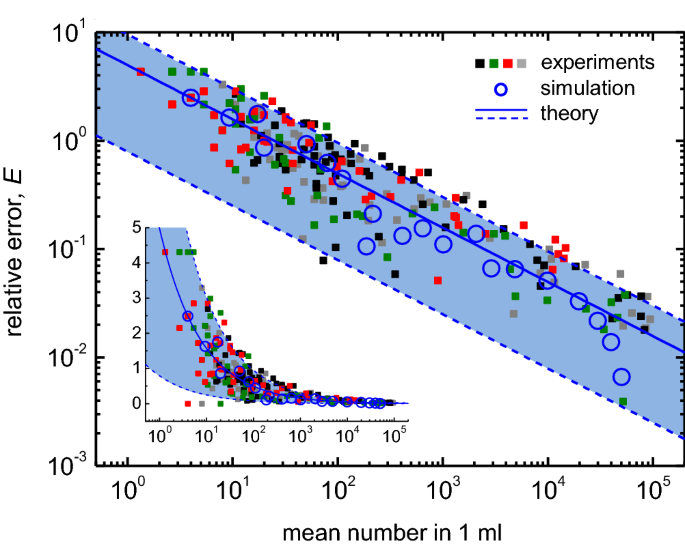# Universal inherent fluctuations in statistical counting of large particles in slurry used for semiconductor manufacturing[ad_1]Figure 3

To quantitatively understand the measurement fluctuation, we derive the probability density function (PDF) for the number of particles in 1 ml. Consider that the average number of large particles in 1 ml of the original slurry is (lambda _0); then, the particle number follows Poisson distribution with average (lambda _0) and standard deviation (sqrt{lambda _0}), as schematically described in Fig. 3a. When diluting the slurry by a factor of (alpha ) and measuring the particle number in (beta ) ml, the PDF for the particle number (x^prime ) again follows Poisson distribution with average ((beta /alpha )lambda _0) and standard deviation (sqrt{(beta /alpha )lambda _0}) (Fig. 3b). Further, a Poisson distribution approaches a normal distribution if the average exceeds 1015; hence, we approximate the PDF for (x^prime ) by a normal distribution with the same average and standard deviation. Then, the particle count (x^prime ) in (beta ) ml is converted to predict the number of particles (x^{prime prime }) in 1 ml of the original slurry, by multiplying by (alpha /beta ). By transforming the random variable (x^prime ), such as (x^{prime prime } =(alpha /beta ) x^{prime } ), we obtain the PDF for (x^{prime prime }) as a normal distribution with average (lambda _0) and standard deviation (sqrt{(alpha /beta )lambda _0}), as described in Fig. 3c. Experimentally, the measurement is repeated several times, and the mean for the expectation value of the particle number in 1 ml is calculated. Let (overline{x^{prime prime }} = (1/n)sum _{i=1}^{n} x^{prime prime }_i) denote the mean of a random sample (x^{prime prime }_i) of size n from the normal distribution for (x^{prime prime }). Then, the PDF of (overline{x^{prime prime }}) is given by a normal distribution with the mean (lambda _0) and standard deviation (sqrt{(alpha /(beta n))lambda _0}), as schematically shown in Fig. 3d.

The PDF for the final mean value (overline{x^{prime prime }}) (Fig. 3d) predicts intrinsic fluctuations, in the order of (sqrt{(alpha /(beta n))lambda _0}), in counting the particles in the slurry. Let us consider the 95 % CI, ([ overline{x^{prime prime }}-d, overline{x^{prime prime }}+d ]) that includes the actual average value (lambda _0), i.e., Pr((overline{x^{prime prime }} -d le lambda _0 le overline{x^{prime prime }} + d)) = 0.95, where d is the margin of error to be determined. Note that Pr((overline{x^{prime prime }} -d le lambda _0 le overline{x^{prime prime }} + d)) = Pr((-d/(s/sqrt{n}) le (overline{x^{prime prime }} – lambda _0)/(s/sqrt{n}) le d/(s/sqrt{n}) )), where s is the standard deviation of a random sample of size n, i.e., (s=(1/(n-1))sum _{i=1}^{n} (x^{prime prime }_i -overline{x^{prime prime }} )). As the variable ((overline{x^{prime prime }} – lambda _0)/(s/sqrt{n})) has a t-distribution with degrees of freedom (n-1), we obtain (d=t_{(0.05/2;n-1)}s/sqrt{n}). Therefore, the relative error E is given by ((t_{(0.05/2;n-1)}s/sqrt{n})/m). As a first approximation, we use (s approx sigma =sqrt{(alpha /(beta n))lambda _0}) and (lambda _0 approx m), which gives

begin{aligned}&E =frac{t_{(0.05/2;n-1)}frac{s}{sqrt{n}}}{m} end{aligned}

(2)

$$approx t_{(0.05/2;n-1)} sqrt{frac{alpha }{beta n}},m^{-0.5}.$$

(3)

Equation (3) shows the inherent fluctuation in the measured value of the mean, which approximately scales with (m^{-0.5}) with a prefactor accounting for the dilution ratio (alpha ), measured volume (beta ,ml), and the number of measurements n in the experiments.Figure 4

The range of the standard deviation of n-measurements, s, provides the upper and lower bounds for E (Eq. (2)). Let [(s_1, s_2)] be an interval where s lies with 95% confidence, i.e., Pr((s_1 le s le s_2))=0.95. Because Pr((s_1 le s le s_2)) = Pr(((n-1)s_1^2/sigma ^2 le (n-1)s^2/sigma ^2 le (n-1)s_2^2/sigma ^2)) and the random variable ((n-1)s^2/sigma ^2) has a (chi ^2)-distribution, we obtain (s_1 = sqrt{chi ^2_{(0.975;n-1)}{alpha }/(beta (n-1))},lambda _0^{+0.5}) and (s_2 = sqrt{chi ^2_{(0.025;n-1)}{alpha }/(beta (n-1))},lambda _0^{+0.5}). The interval [(s_1, s_2)] determines the 95% CI for E, as follows.

$$E_{mathrm{low}} = t_{(0.05/2;n-1)}sqrt{chi ^2_{(0.975;n-1)}frac{alpha }{ (n-1) n beta }},m^{-0.5},$$

(4)

$$E_{mathrm{high}} = t_{(0.05/2;n-1)}sqrt{chi ^2_{(0.025;n-1)}frac{alpha }{ (n-1) n beta }},m^{-0.5},$$

(5)

where we have used (lambda _0 approx m). One may derive the exact distribution function for the relative error E based on the probability density function of the coefficient of variation21,22. However, the empirical approximation, Eqs. (4,5), provide good estimates for the upper and lower bounds of experimentally measured E defined by Eq. (1), respectively, and both Eqs. (4,5) converge to Eq. (3) as (n rightarrow infty ).

The experimentally measured relative error E is in good agreement with the theoretical prediction, Eqs. (4,5) as well as the Monte Carlo simulation, as shown in Fig. 4. Each colored, filled dot (black, green, red, and gray) in Fig. 4 corresponds to the E shown in Fig. 2c and is plotted as a function of the measured mean m. Overall, the experimentally obtained relative errors excellently scale with (m^{-0.5}), following the blue solid curve (Eq. (3)), and they mostly lie between the lower and upper bounds indicated by the blue dashed curves (Eqs. (4,5)). A Monte Carlo simulation was performed to simulate particle counts in a given sample volume with different mean numbers, which results in the relative errors, denoted by the blue empty circles in Fig. 4. Remarkably, the upper and lower bounds (Eqs. (4,5)) quantitatively predicts highly fluctuating data at a low mean number, as observed in the experiments (inset of Fig. 4). As the particle number decreases with increasing particle diameter for the slurry investigated herein (Fig. 2a), the seemingly higher E at bigger particles (Fig. 2b) is due to the fact that the number of bigger particles is lower than that of smaller particles.

[ad_2]

Source link